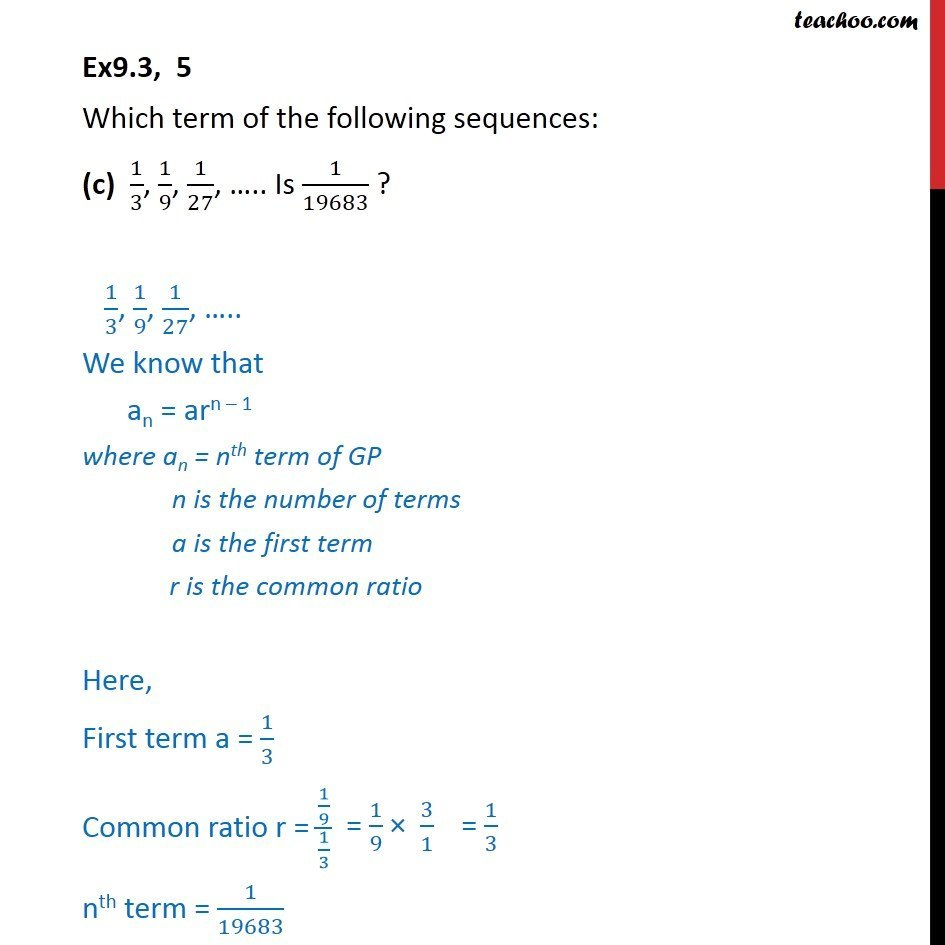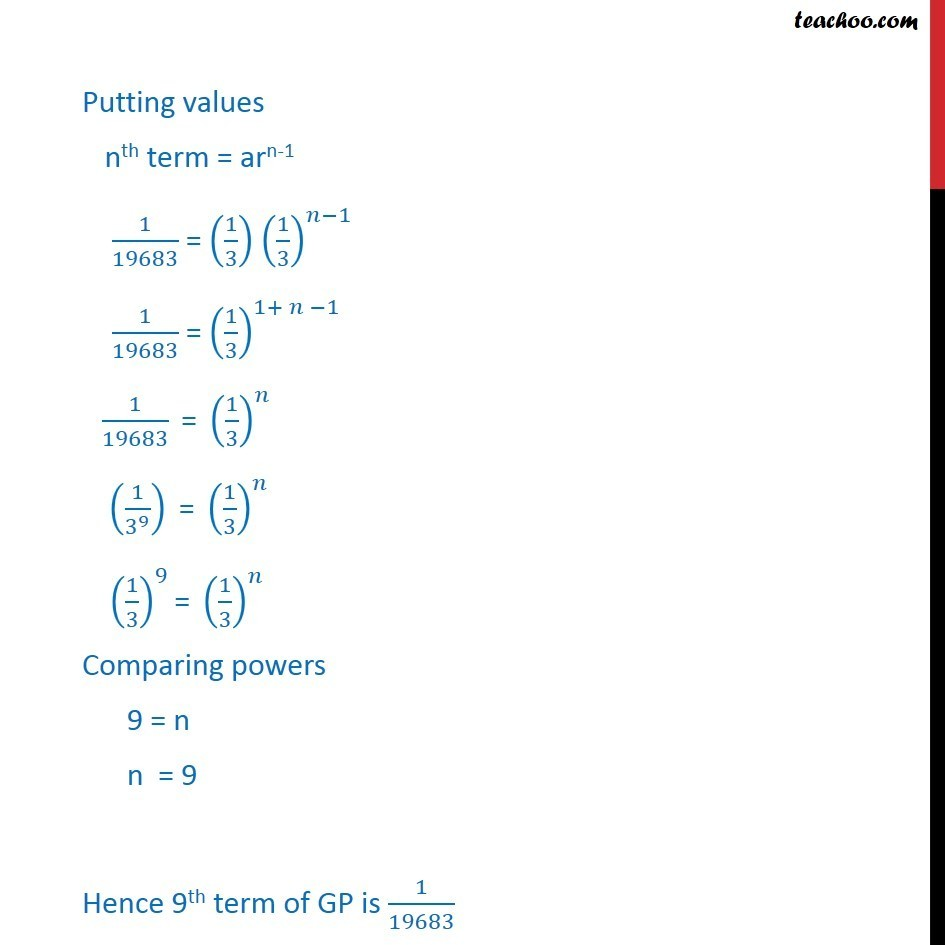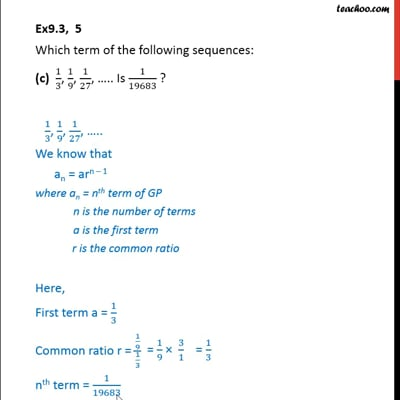Ex 9.3

Chapter 9 Class 11 Sequences and Series
Serial order wiseThis video is only available for Teachoo black users

Introducing your new favourite teacher - Teachoo Black, at only ₹83 per month

### Transcript

Ex9.3, 5 Which term of the following sequences: (c) 1/3, 1/9, 1/27, .. Is 1/19683 ? 1/3, 1/9, 1/27, .. We know that an = arn 1 where an = nth term of GP n is the number of terms a is the first term r is the common ratio Here, First term a = 1/3 Common ratio r = (1/9)/(1/3) nth term = 1/19683 Putting values nth term = arn-1 1/19683 = (1/3) (1/3)^( 1) 1/19683 = (1/3)^(1+ 1) " " 1/19683 " = " (1/3)^ " " (1/3^9 )" = " (1/3)^ " " (1/3)^9 "= " (1/3)^ Comparing powers 9 = n n = 9 Hence 9th term of GP is 1/19683